# Two-Variable Data Table In ExcelArticle byExcelMojo Team## What Is Two-Variable Data table In Excel?

The Two-Variable Data Table in Excel is a generated data table using the What-if analysis. It shows the impact of two input variables provided simultaneously as row and column values on a formula outcome. In this data table, we can calculate multiple formulas at once.

Users can use the two-variable data table when comparing different loan schemes or projecting the annual company sales based on expenses and growth.

For example, the first table below shows a line equation and the different parameters in the equation.

We can find the y-value in cell C7 as 5, with the values of m, x, and c,

The second table is the two-variable data table, formed using two variables, the slope, m-values, in cell F2:H2, and x-coordinates in cell E3:E5. And cell E2 contains the line equation.

We must calculate the y-coordinates and fill the values in cells F3:H5. Then select the required cell range and use the DataWhat-If AnalysisData Table to update the two-variable data table with the required y-coordinates.

We get the generated Two-Variable Data Table in Excel, as shown above.

[Output Observation: The Data Table feature takes the cell E2 equation as the formula to determine and update the y-values in the required target cells. Thus, the cell E2 formula is crucial.

In cell F3, the calculated y-coordinate is 7. When we use the Data Table excel option, the line equation in cell F3 gets substituted with the m-value as 2 (cell F2)and the x-value as 2 (cell E3). So, the equation in cell F3 becomes: y = 2 * 2 + 3 = 7, where the c-value remains as 3.]

###### Key Takeaways
• The Two-Variable Data Table in Excel helps analyze a formula output based on the changes made to the values of two variables simultaneously.
• This data table helps users compare different loan schemes and determine the future value of an investment.
• To create a two-variable data table, first, we need to enter the required equation value, with the two input variables in question, in the first cell of the cell range. And the first row and column of the target cell range will have the two input variable values. Then we should select the target cell range to generate the data table.
• We can take the input and output from one or multiple worksheets.

### How to Create Two-Variable Data Table in Excel?

We can create Two-Variable Data Table as follows:

• First, ensure the source data contains the precise formula with the two input variables required to create the data table.
• Next, select the data table range → select the “Data” tab → go to the “Forecast” group → click the “What-If Analysisoption drop-down → select the “Data Table” option, as shown.
• The “Data table” dialog box pops up. Enter the references in the “Row input cell: and Column input cell:” fields to create the required data table → click “OK”, as shown below, to view the formula output or the generated data table based on the values of the two input variables.

Let us take an example to understand this.

Here, we will create a Two-Variable Data Table in Excel for the following example.

The first table shows the parameters required to calculate the compound interest (CI), and the calculated CI value in cell B9.

The steps to create the Two-Variable Data Table in Excel are,

1. Let’s do the basic checks first, the given CI formula in cell E3 is correct, the given year values are 5, 6, and 7, and the given initial investment values are \$6k to \$10k. Also, the target cells F4:H8 data format is Currency.

Choose cell range, E3:H8 → select the “Data” tab → go to the “Forecast” group → click the “What-If Analysis” option drop-down → select the “Data Table” option, as shown below.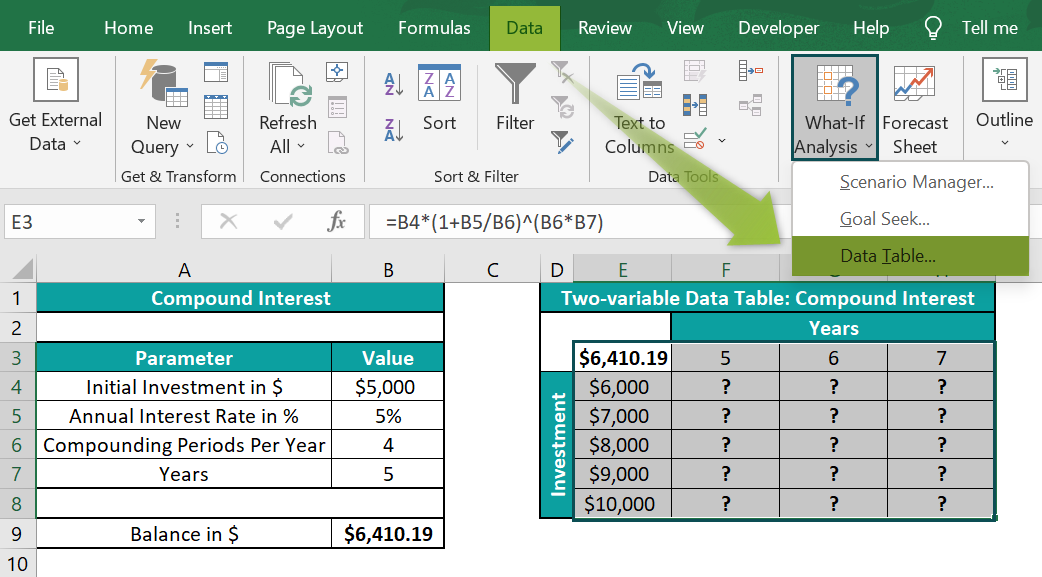2. In the “Data Table” dialog box that pops up, enter the row and column input cells B7 and B4, respectively, for years and initial investment value, as shown below.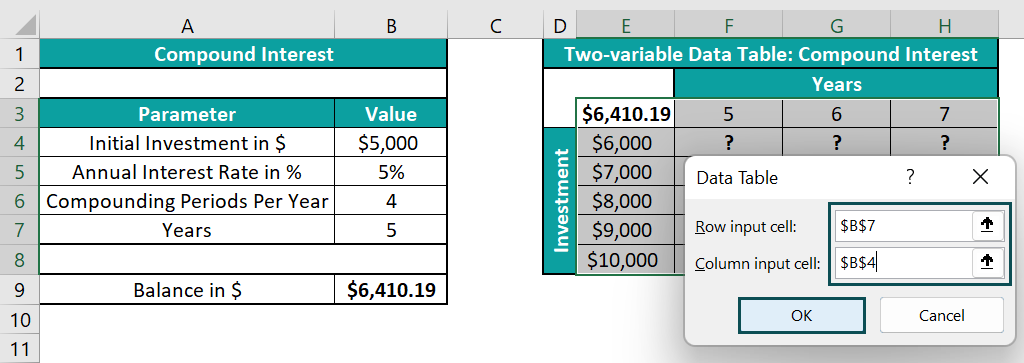3. Click “OK” in the Data Table window to view the calculated CI values in cells F4:H8.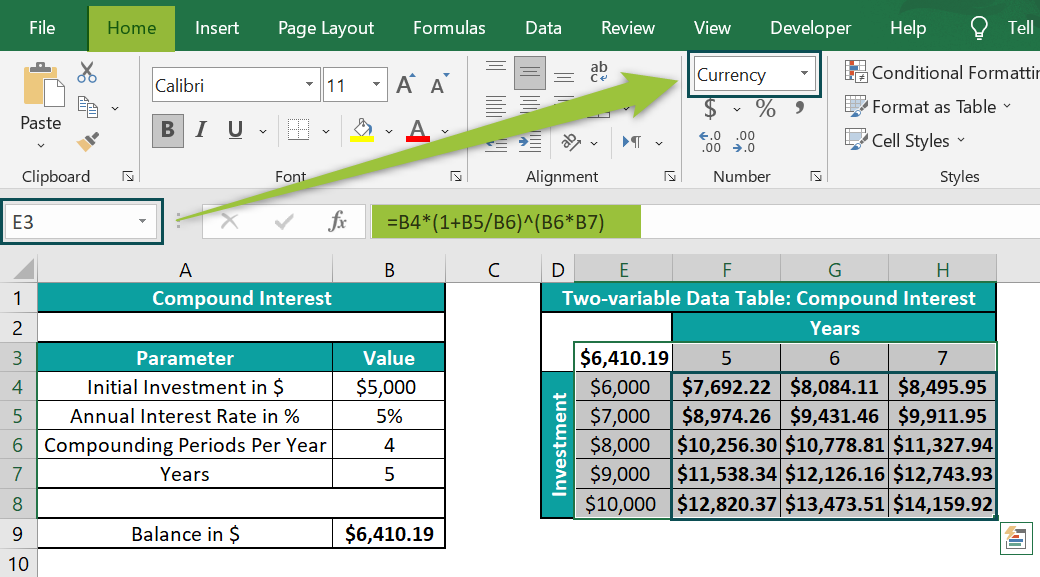We will get the generated Two-Variable Data Table, as shown above.

[Output Observation: Let us consider cell F4 value to see how the feature works. The data table takes cell E3 formula and substitutes the initial investment as \$6,000 (cell E4), the annual interest rate as 5%, and the compounding periods per year as 4. And it takes the years as 5 (cell F3) to return the calculated CI value as \$7,692.22.
Likewise, in the case of cell G6 value, the formula takes the initial investment as \$8,000 and years as 6. And with the annual interest rate and compounding periods per year remaining the same, the calculated CI value in cell G6 is \$10,778.81.]

### Examples

We will understand some advanced scenarios with the Two-Variable Data Table in Excel examples.

#### Example #1

The below images show tables in two worksheets from the same workbook, namely, Two-var_Calculation Sheet and Two-var Output Sheet.

The steps to create a Two-Variable Data Table from multiple worksheets are,

• 1: First, we shall create dummy variables in both sheets. In the case of the Two-var Output Sheet, the dummy variables are the loan amount and the number of monthly payments. And their corresponding cells B3:B4 should be empty.

On the other hand, enter a dummy value using the IF() for each input variable in the Two-var_Calculation Sheet.

[The two IF()s in the first sheet check whether the cells B3 and B4 in the second sheet are empty. If they are, the loan amount and the number of monthly payment values are the cells B4 and B6 values, respectively. Otherwise, they will take the values for the two variables given in the Two-var Output Sheet, the second sheet.]

• 2: Select cell E3, enter the formula =PMT(10%/12,’Two-var_Calculation Sheet’!C6,’Two-var_Calculation Sheet’!C4), and pressEnter”.

[It will connect the data table in the Two-var Output Sheet with the dummy loan amount and number of monthly payments in the first sheet.]

• 3: Select the cells E3:H8 à select the “Data” tab → go to the “Forecast” group → click the “What-If Analysis” option drop-down → select the “Data Table” option, as shown below.
• 4: In the “Data Table” dialog box that pops up, enter the row and column input cells B3 and B4, respectively.
• 5: Click “OK” in the Data Table window to view values in cells F4:H8.

We get the generated Two-Variable Data Table, as shown above.

[Output Observation: Let us see cell H8 value to understand how the feature works across two worksheets. The IF excel function uses the dummy variables to link the data table in the second sheet with the source table in the first sheet. It ensures the input variables always have values. In cell H8, the dummy loan amount and number of monthly payments are \$40,000 and 110, respectively. And the data table uses these values in the PMT excel funciton to return the monthly payment amount as -\$556.83, an outgoing value.]

#### Example #2

We will create a Two-Variable Data Table in Excel to calculate an investment’s future value for the given payment terms and annual interest rates.

The first table, shown below, contains the payment information, and the second table is where we need to build the two-variable data table for the given payment terms, 15, 20, and 25, and annual interest rates of 6% to 10%.

• 1: Ensure cell E3 contains a reference to the formula provided in cell B8, i.e., =FV(B7/12,B5*B6,-B4), and has the data format set as Currency.
• 2: Select cells E3:H8 → select the “Data” tab → go to the “Forecast” group → click the “What-If Analysis” option drop-down → select the “Data Table” option, as shown below.
• 3: In the “Data Table” dialog box that pops up, enter the row and column input cells B5 and B7, respectively.
• 4: Click “OK” in the Data Table window to view values in cells E3:H8.

We get the generated Two-Variable Data Table in Excel, as shown above.

[Output Observation: For example, in the case of cell H8, while the rest of the parameters will have the same values, the payment term will be 25 years, and the annual interest rate will be 10%. And hence it shows the calculated future value as \$6,634,167.01.]

### Uses of Two-variable Data Table in Excel

The uses of the Two-Variable Data Table in Excel are:

• It helps determine how two inputs can change a single output.
• The Data Table feature can help assess aspects such as loan payments and an investment’s future value.

### Important Things to Note

• We cannot delete or modify a cell value from the Two-Variable Data Table, as it is an array formula in excel. Therefore, we must delete the entire data table to modify the data.
• We cannot undo an action performed in the Two-Variable Data Table in Excel.
• The data table works like a linked formula. And thus, it does not require manual updating.

1. What option should we use to create a Two-Variable Data Table in Excel?

To create a Two-Variable Data Table in Excel, choose an empty cell range for the output → select the “Data” tab → go to the “Forecast” group → click the “What-If Analysis” option drop-down → select the “Data Table” option, as shown below.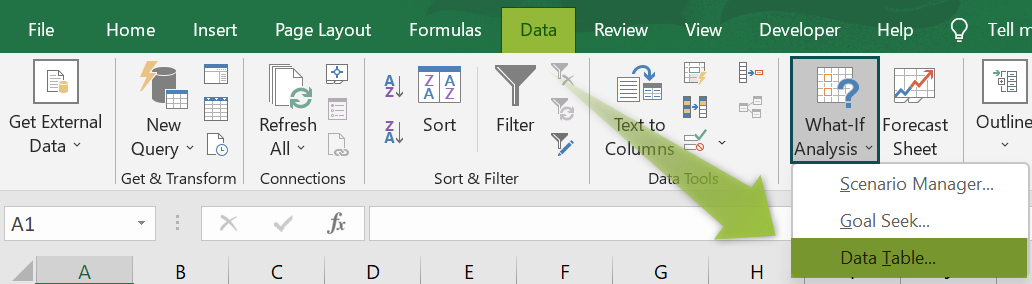2. Can we create a Two-Variable Data Table for the projected sales of a specific year, given the year’s growth and expenses?

We can create a Two-Variable Data Table for the projected sales of a specific year, given the year’s growth and expenses.

For instance, consider the two-variable data table for the projected sales of 2021, given the 2021 growth and expenses.

The below image shows two tables. The first table contains the sales projection calculation for 2021. And cell B5 contains the formula to determine the 2021 sales projection from the given values in cells B2:B4.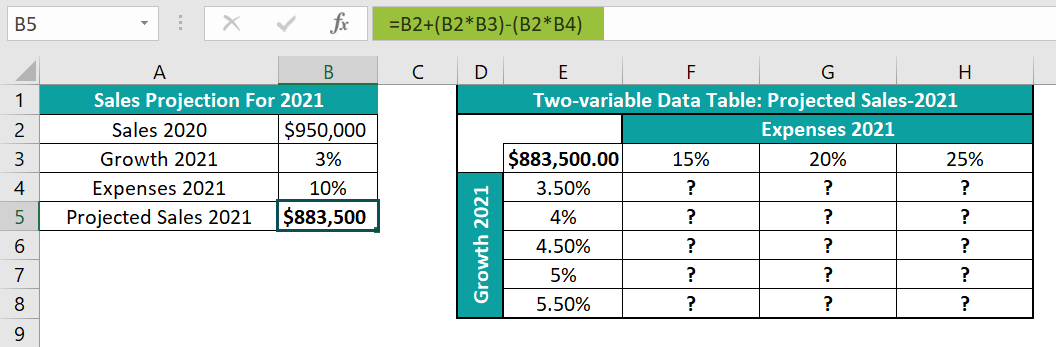The steps to create a Two-Variable Data Table in Excel are,

• 1: Ensure cell E3 contains reference to the the formula in cell B5, i.e., =B2+(B2*B3)-(B2*B4).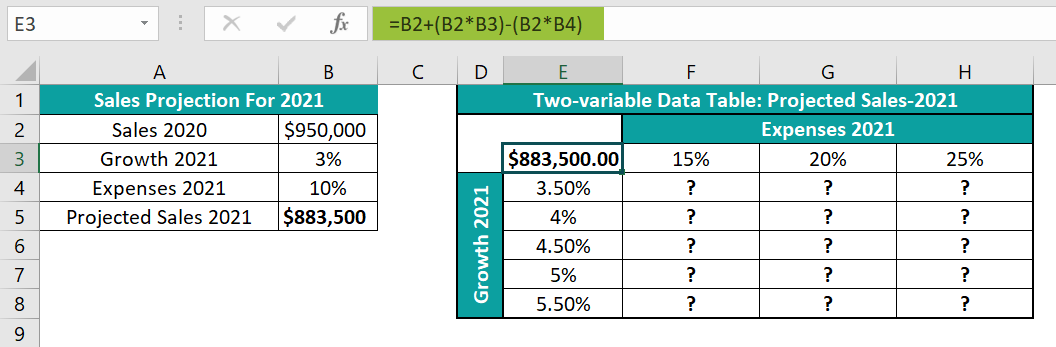• 2: Select cells E3:H8 → select the “Data” tab → go to the “Forecast” group → click the “What-If Analysis” option drop-down → select the “Data Table” option, as shown below.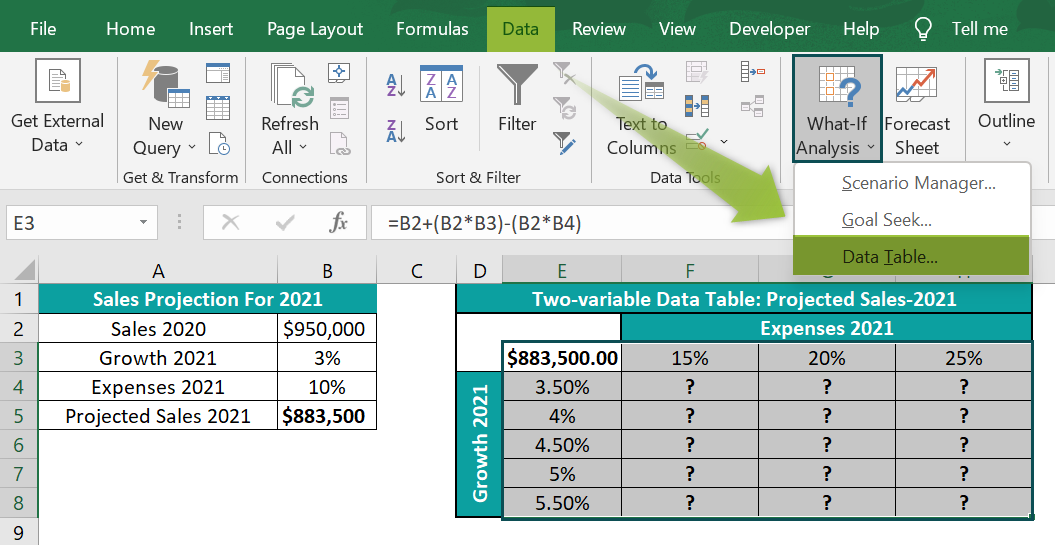• 3: In the “Data Table” dialog box that pops up, enter the row and column input cells B4 and B3, respectively.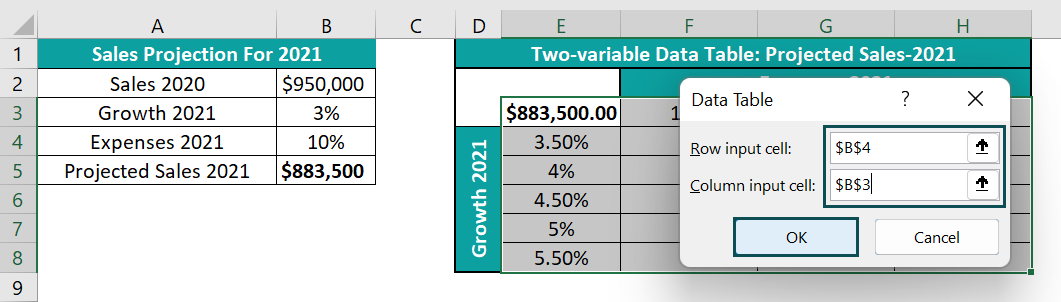• 4: Click “OK” in the Data Table window to view values in cells F4:H8.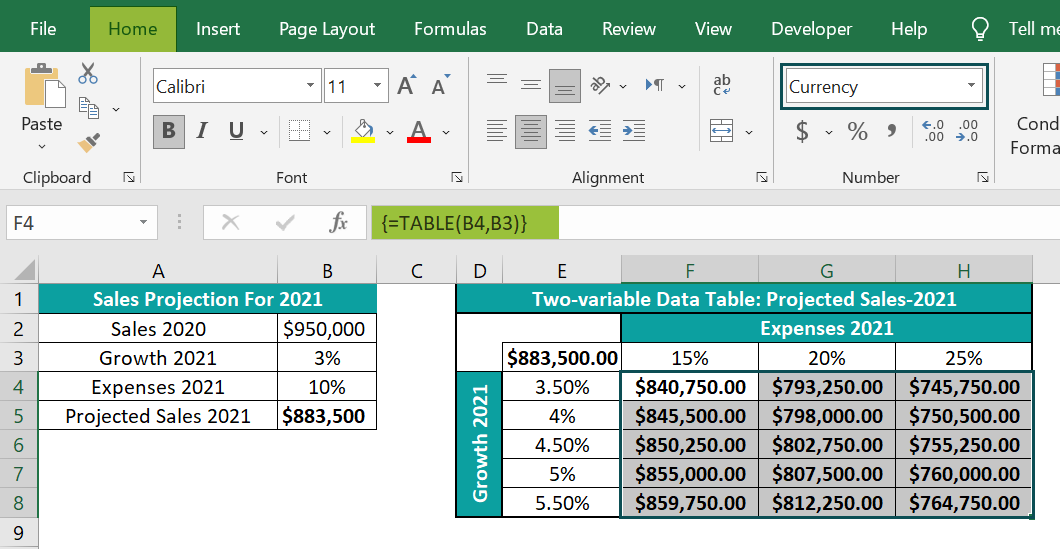We get the generated Two-Variable Data Table in Excel, as shown above.
Thus, we get the 2021 sales projections for the various input values of 2021 expenses and growth percentages.

3. Why is my two-variable data table not working in Excel?

Our two-variable data table is not working in Excel, perhaps due to the following reasons:
The cells range for the generated data table is not selected as an array.
We did not correctly define the two input variables, row, and column values, which we must change simultaneously to analyze the specified formula output.